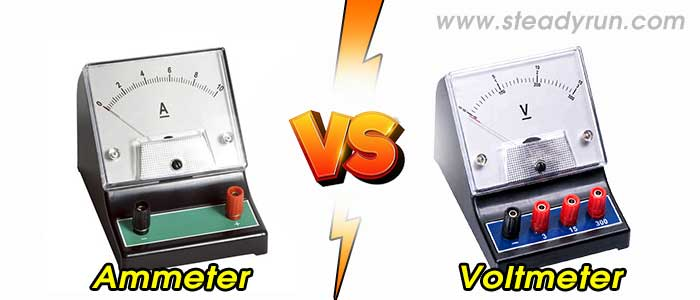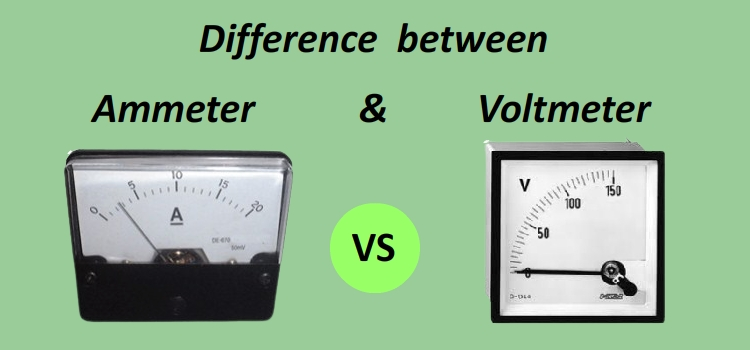# Differences between Ammeter and VoltmeterDistinguish, differentiate, compare and explain what is the differences between Ammeter and Voltmeter. Comparison and Difference.

### What is Ammeter and Voltmeter?

Ammeter – It is an instrument which is used to measure the current in an electrical circuit. It gets its name from Ampere which is the unit of electric current.

Voltmeter – This instrument measures the voltage between two points in an electrical circuit. It is present in both, digital and analogue form.## Differences between Ammeter and Voltmeter

1. Ammeter is a low resistance instrument. Voltmeter is a high resistance instrument.

2. In ammeter, resistance is GS / (G + S). In voltmeter, resistance is G + R .

3. In ammeter, Shunt Resistance is (G lg) / (l - lg) and is very small. In voltmeter, series Resistance is (V / lg) - G and is very high.

4. Ammeter is always connected in series. Voltmeter is always connected in parallel.

5. Resistance of an ideal ammeter is zero. Resistance of an ideal voltmeter is infinity.

6. In the first one, Its resistance is less than that of the galvanometer. In the second one, its resistance is greater than that of the voltmeter.

7. It is not possible to decrease the range of the given ammeter. It is possible to decrease the range of the given voltmeter.

#### Ammeter

Ammeter is employed for measuring of current in a circuit and connected in series in the circuit. As ammeter is connected in series, the voltage drop across ammeter terminals is very low. This requires that the resistance of the ammeter should be as low as possible. The current coil of ammeter has low current carrying capacity whereas the current to be measured may be quite high. So for protecting the equipment a low resistance is connected in parallel to the current coil and it is known as shunt resistance.
Ideal ammeter should have zero resistance.

#### Voltmeter

Voltmeter is employed to measure the potential difference across any two points of a circuit. It is connected in the parallel across any element in the circuit. The resistance of voltmeter is kept very high by connecting a high resistance in series of the voltmeter with the current coil of the instrument. The actual voltage drop across the current coil of the voltmeter is only a fraction of the total voltage applied across the voltmeter which is to be measured.
Ideal voltmeter should have infinite resistance.

#### Tags:

Difference between Voltmeter vs Ammeter

Ammeter vs Voltmeter

Differences between Voltmeter vs Ammeter

### Latest Articles### Spreading Knowledge Across the World

USA - United States of America  Canada  United Kingdom  Australia  New Zealand  South America  Brazil  Portugal  Netherland  South Africa  Ethiopia  Zambia  Singapore  Malaysia  India  China  UAE - Saudi Arabia  Qatar  Oman  Kuwait  Bahrain  Dubai  Israil  England  Scotland  Norway  Ireland  Denmark  France  Spain  Poland  and  many more....